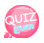# control statement - switch

The if statement selects either true or false, while the switch selects one of several.
To use a switch, find and select the value of the variable declared as true among the various cases given.
If the variable \$a = 3 is declared, the corresponding case is selected and the process for executing the statement is as follows.

```<?php
echo "Switch statement when declaring \\$a = 2<br />";

\$a = 2;

switch(\$a) {
case 0:
echo "The variable \ \$a is the number 0 ....<b> case 0:</b><br />";
break;

case 1:
echo "The variable \\$a is the number 1 ...<b> case 1:</b><br />";
break;

case 2:
echo "The variable \\$a is the number 2 .... <b> case 2:</b><br />";
break;

case 3:
echo "The variable \\$a is the number 3 ... <b> case 3:</b><br />";
break;

case 4:
echo "The variable \\$a is the number 4 ... <b> case 4:</b><br />";
break;

default:
echo "The variable \\$a = {\$a} is a number other than 0,1,2,3.<br />";
}
?>```Assigning 2 as the value of \$a and setting \$a as the condition of the switch,
So if you specify case 1, case 2, case 3 case 4
The matched switch is 2, so the execution statements in case 2 are executed.
There is a break in each case statement, which executes the above statement when the condition is met and exits the switch statement when a break statement is encountered.
If you don't declare break you get the following result:

```<?php
echo "Switch statement when declaring \\$a = 2<br />";

\$a = 2;

switch(\$a) {
case 0:
echo "The variable \ \$a is the number 0 ....<b> case 0:</b><br />";

case 1:
echo "The variable \\$a is the number 1 ...<b> case 1:</b><br />";

case 2:
echo "The variable \\$a is the number 2 .... <b> case 2:</b><br />";

case 3:
echo "The variable \\$a is the number 3 ... <b> case 3:</b><br />";

case 4:
echo "The variable \\$a is the number 4 ... <b> case 4:</b><br />";

default:
echo "The variable \\$a = {\$a} is a number other than 0,1,2,3.<br />";
}
?>```Since the break statement is not declared, the following cases are executed without exiting after satisfying the condition of 2.
If the above example is implemented as an if statement, it is as follows.

```<?php
echo "Convert switch statement to if statement<br />";
echo "<hr />";

\$a = 3;

if(\$a == 0)
echo "The variable \$a is the number 0<br>";
else if(\$a == 1)
echo "The variable \$a is the number 1<br>";
else if(\$a == 2)
echo "The variable \$a is the number 2<br>";
else if(\$a == 3)
echo "The variable \$a is the number 3<br>";
else
echo "0, 1, 2, 3 are not all.";
?>```The following is the use of switch statement.

```<?php
echo "Display month's date with switch statement<br /><hr />";

\$mon = 2;

switch(\$mon) {
case 1:
case 3:
case 5:
case 7:
case 8:
case 10:
case 12:
echo "The {\$mon} month has 31 days.<br />";
break;

case 4:
case 6:
case 9:
case 11:
echo "Month {\$mon} has 30 days<br />";
break;

case 2:
echo "{\$mon} months have 28 days in the previous year.<br />";
break;

default:
echo "{\$mon} is a number other than 1-12, representing the month.<br />";
}
?>```The switch statement can also be used as a condition such as big or small.
Like the following example.

```<?php
echo "Put condition of switch statement<br /><hr />";

\$age = 23;

switch(\$age) {
case(\$age >= 10 && \$age <= 19):
echo "I am a teenager.";
break;

case(\$age >= 20 && \$age <= 29):
echo "I'm in my twenties.";
break;

case(\$age >= 30 && \$age <= 39):
echo "I am in my 30s.";
break;

case(\$age >= 40 && \$age <= 49):
echo "I am in my 40s.";
break;

case(\$age >= 50 && \$age <= 59):
echo "I am in my 50s.";
break;

case(\$age >= 60 && \$age <= 69):
echo "I am in my 60s.";
break;

case(\$age >= 70 && \$age <= 79):
echo "I am in my 70s.";
break;

case(\$age >= 80 && \$age <= 89):
echo "I am in my 80s.";
break;

case(\$age >= 90 && \$age <= 99):
echo "I am in my 90s.";
break;

default:
echo "you are baby PHPer";
}
?>```